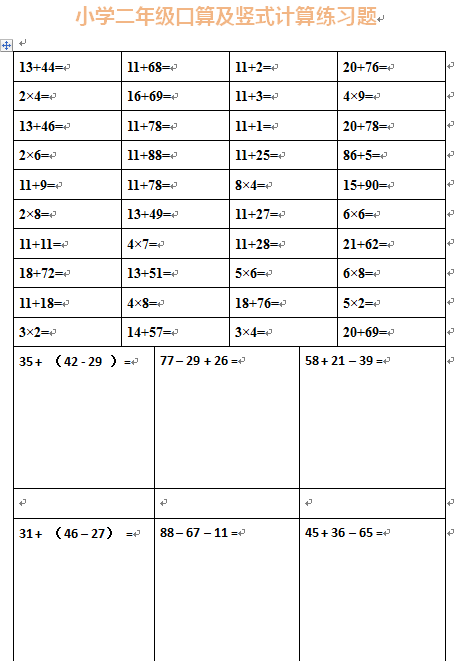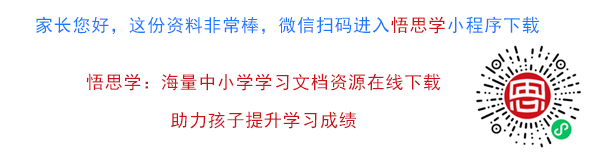13+44= 11+68= 11+2= 20+76= 2×4= 16+69= 11+3= 4×9= 13+46= 11+78= 11+1= 20+78=

【DOC文档28页】小学二年级下册数学口算及竖式计算练习题A4电子版资料_可直接打印_会员免费下载##### 评论信息